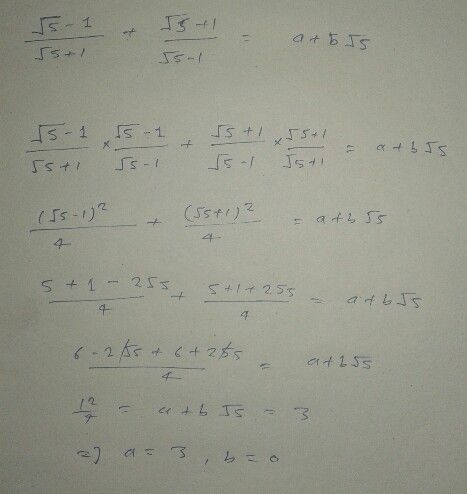Symbol
Problem$a$ $\dfrac {\sqrt{5} -1} {\sqrt{5} +1}+\dfrac {\sqrt{5} +1} {\sqrt{5} -1}=a+b\sqrt{5}$
Other
SolutionQanda teacher - Nitin Sing